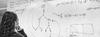# 1st Semester 2017/18: Alternative Set Theories

Instructors
Yurii Khomskii [The project is coordinated at the ILLC by Benedikt Lowe]
ECTS
6
Description
The generally accepted foundational theory for all of mathematics is ZFC (Zermelo-Fraenkel axioms with the Axiom of Choice). ZFC is a first-order theory which uses a single non-logical relation symbol (set membership), and an infinite but recursive collection of axioms postulating the existence of various sets.

Throughout the course of the 20th century, many alternative systems of "set theory" have been proposed. Some of these were conceived as improvements of ZFC; some as alternative conceptions with a more pleasant philosophical justification; others aiming at specific applications, and yet others simply out of curiosity. One can alter ZFC in a number of ways: by choosing a different collection of axioms (leaving some out or adding stronger ones), by changing the logical ontology (what counts as formal object, which relation symbols are used), by changing the underlying logic (e.g., intuitionistic instead of classical logic), or a combination of the above.

In this project, we plan to look at some of these alternative set theories. Based on the preference of the students, we will choose to study some or all of the following alternative systems:

1. NGB (von Neumann-Gödel-Bernays) and MK (Morse-Kelley) class theories. These theories treat classes as formal objects of the language, distinct from sets. The appealing feature is a "class comprehension schema", stating that for every (suitable) sentence phi there is a class of all objects satisfying phi.
2. KP (Kripke-Platek). This theory is considerably weaker than ZFC, obtained by removing the "non-predicative" elements of standard set theory. It has connections to generalised recursion theory and the theory of admissible ordinals.
3. NF (Quine's New Foundations). First proposed by Quine in 1937 as a simplification of the type theory from Russell's Principia Mathematica, this theory has intuitive philosophical underpinnings. Its only axioms are extensionality and naive comprehension for stratified formulas phi, where a formula is "stratified", if, roughly speaking, the variables occurring in it can be assigned "types" in a consistent way. For example "x \in y" is stratified, whereas "x \in x" is not stratified. One fascinating aspect of NF is that its consistency has remained a fundamental open problem until very recently (Randall Holmes, 2010).
4. IZF and CZF (Intuitionistic Set Theory and Constructive Set Theory). Here, classical logic is replaced by intuitionistic logic. In the former case, the ZFC axioms are left intact, aside of those that would imply Excluded Middle; in the latter case, the axioms themselves are fundamentally altered.
5. Non-wellfounded set theory, particularly the Anti-foundation Axiom AFA of Peter Aczel. In this set theory every "system of equation" has a "solution". For example, there exists a set x satisfying the equation "{x} = x", and there exist sets x and y satisfying the equation system "x = {y}" and "y = {x}". This type of set theory has been claimed to have applications in computer science.
6. Set Theory based on other logics (e.g., paraconsistent set theory, modal set theory). This is an experimental direction since there is no established literature.
Organisation
Each student will choose a topic and give a presentation of 2 hours. In the first (preliminary) meeting, we will start by giving an overview of the various alternative set theories mentioned above, followed by a distribution of topics to students. The exact content of the topics will be determined during that meeting.
Prerequisites
Basic knowledge of set theory and logic (some of the topics require more advanced knowledge).
Assessment
Each student will need to hand in a written report, of approximately 3 pages, at the end of the project. This report, together with the presentation, will be evaluated on a "pass/fail" basis.